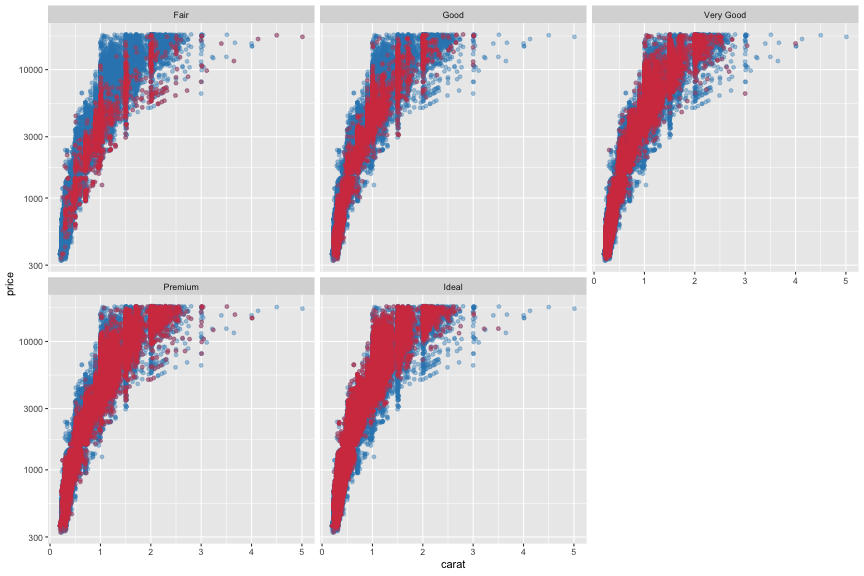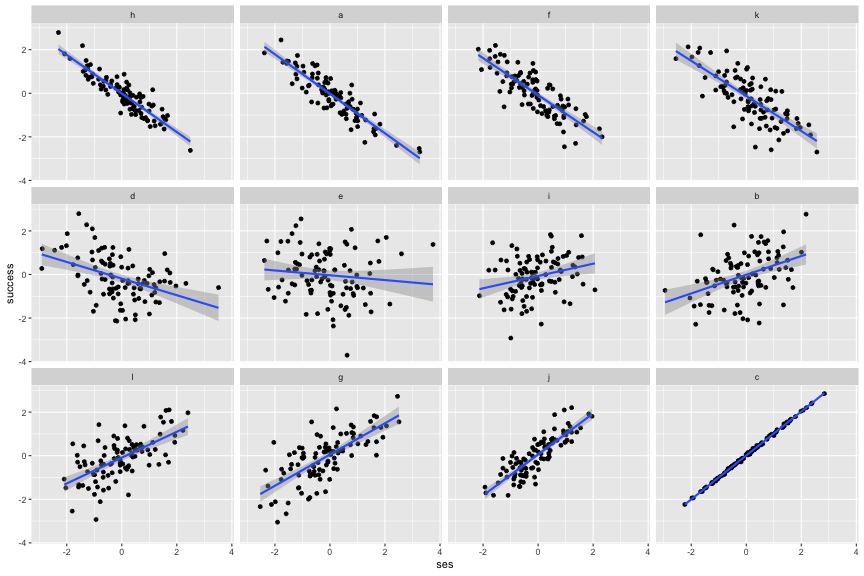## Introduction

Today I read an interesting post on R-Bloggers. In the post, Method Matters outlines a solution to a typical data scientist interview problem: FizzBuzz.

```In pseudo-code or whatever language you would like: write a program that prints the numbers from 1 to 100. But for multiples of three print “Fizz” instead of the number and for the multiples of five print “Buzz”. For numbers which are multiples of both three and five print “FizzBuzz”.
```

The problem probes your knowledge of basic programming concepts. More specifically, it asks: “Are you able to identify and deal with multiple cases?” Method Matters introduces two simple solutions in R and Python. The first solution uses a for-loop. It iterates over a set of if-else-statements. I take no issue with this approach even though more elegant implementations are possible. The second solution uses a function to do the heavy lifting, which strikes me as more interesting, more powerful, and more questionable given its implementation by Method Matters.

## 99 problems but for() ain’t one

Method Matters defines a function which takes a numeric input to pass through the set of if-else-statements defined in the first solution. It exemplifies how working code can be recycled in more complex settings. Take a moment to study the original code, I’ll detail my reservations below.

``````# define the function
fizz_buzz <- function(number_sequence_f){
if(number_sequence_f%%3 == 0 & number_sequence_f%%5 == 0) {
print('FizzBuzz')
}
else if(number_sequence_f%%3 == 0) {
print('Fizz')
}
else if (number_sequence_f%%5 == 0){
print('Buzz')
}
else {
print(number_sequence_f)
}

}

# apply it to the numbers 1 to 100
sapply(seq(from = 1, to = 100, by = 1), fizz_buzz)
``````

So what’s not to like about it? Two things bug me in particular.

1. The function does not abstract from the particular instance of the problem. Note how values 3, 5, Fizz, Buzz, and FizzBuzz have been hard-coded into the function’s body. Given that the function recycles a for-loop this is not surprising. However, functions (should) encapsulate algorithms which solve entire classes of problems.
2. More importantly, the function is not vectorized. In R, control statements such as if() or while() expect logical vectors of length one. Whatever condition you pass to them must evaluate to a single Boolean statement.1 Since `fizz_buzz()` consists entirely of such statements, it expects you to pass each sequence element individually. The concluding call to `sapply()` does just that. I don’t mean to offend, but `sapply(seq(from = 1, to = 100, by = 1), fizz_buzz)` is `for()` in disguise.

## fizz_buzz() refactored

The refactored solution shown below generalizes from FizzBuzz, and it is vectorized. The function expects two arguments: (1) a numeric vector such as an integer sequence from 1 to 100, and (2) a “dictionary” of named values to evaluate, e.g., `c("Fizz" = 3, "Buzz" = 5)`. The first argument is checked for multiples of each entry in the dictionary. Those TRUE/FALSE evaluations are saved in a logical matrix with rows equal to `length(x)` and columns equal to `length(dictionary)`. Next, each column of that matrix is used to logically index the argument `<x>` and its selected elements are replaced by the corresponding name from the dictionary. Finally, the function returns a vector of strings.

``````replace_sequence_elements <- function(x, dictionary){
# The function searches <x> for multiples of each element
# in <dictionary>. Multiples are replaced by the name of
# the corresponding dictionary element. If some element
# of <x> is a multiple of all elements in dictionary,
# then it will be replaced by concatenated dictionary
# names.
# x ... numeric vector
# dictionary ... named, numeric vector
# The function returns a vector of type character.
stopifnot("names" %in% names(attributes(dictionary)))

out <- as.character(x)
K <- length(dictionary)
tests <- matrix(
FALSE, nrow = length(x), ncol = length(dictionary)
)

for(k in seq(K)) {
tests[, k] <- x %% dictionary[k] == 0
out[tests[, k]] <- names(dictionary[k])
}
out[rowSums(tests) == K] <- paste(
names(dictionary), collapse = ""
)

return(out)
}
``````

Let’s see if this works:

``````replace_sequence_elements(seq(15), c("Fizz" = 3, "Buzz" = 5))
``````
```##   "1"        "2"        "Fizz"     "4"        "Buzz"     "Fizz"
##   "7"        "8"        "Fizz"     "Buzz"     "11"       "Fizz"
##  "13"       "14"       "FizzBuzz"
```

## Conclusion

This post responds to and refactors a publicly available solution of a common data scientist interview problem: FizzBuzz. The refactored solution generalizes from the original and can now take any number of cases to evaluate. Moreover, it is vectorized. That said, several pain points remain. For instance, a for-loop is still doing the heavy lifting. As the number of test cases increases this may cause performance issues, at least in R. Also, the refactored solution makes specific assumptions about the structure of its arguments. There must be no missing elements, and test cases must be passed in the form of a named vector. A different approach would be needed to avoid or at least reduce the number of such implicit assumptions. Two instructive examples may be found in the comments to Method Matters’ original post.

## Introduction

This post demonstrates how small multiples can be used to highlight different parts of a distribution. In principle, ggplot2 offers many, easy to use options that partial out different groups in your data. The aesthetics color, size, and shape come to mind. Moreover, the purpose of small multiples is to show the same (bivariate) association across different groups in your data. Notwithstanding, either approach has drawbacks. When mapping categories to an aesthetic like color, all groups remain on the same canvas. The result may be wanting, especially when you work with big datasets. Small multiples, in contrast, draw out each group but deemphasize the grand picture. Wouldn’t it be nice to find some middle ground?

## Motivation

We are going to work with the diamonds data which is available from the ggplot2 package. The goal is to highlight each cut in a scatter plot of price against carat without falling into either of the extremes mentioned above. Here is what the data look like:

``````rm(list = ls())
library("tidyverse")
data(diamonds)
select(diamonds, price, carat, cut)
``````
```## # A tibble: 53,940 x 3
##    price carat cut
##    <int> <dbl> <ord>
##  1   326 0.23  Ideal
##  3   327 0.23  Good
##  5   335 0.31  Good
##  6   336 0.24  Very Good
##  7   336 0.24  Very Good
##  8   337 0.26  Very Good
##  9   337 0.22  Fair
## 10   338 0.23  Very Good
## # … with 53,930 more rows
```

## Recipe

As always, smart layering is the answer. We are going to plot the diamonds data twice using different colors: Once for all diamonds in the data, and once for each cut. The code also includes minor finishing touches (opacity and color).

``````alpha_lvl <- .4
ggplot(data = diamonds, aes(x = carat, y = price)) +
geom_point(
data = select(diamonds, -cut),
# Dropping <cut> plots all our data.
colour = "#3288bd", alpha = alpha_lvl
) +
geom_point(colour = "#d53e4f", alpha = alpha_lvl) +
scale_y_log10() +
facet_wrap(vars(cut))
``````## Conclusion

This post demonstrates how small multiples can highlight different segments within a distribution without losing sight of its overall shape. The key is smart layering. Plot the data twice: Once ignore and once highlight your facets.

## Introduction

This post will show you how to order small multiples in ggplot2 by arbitrary criteria. It is straightforward to generate small multiples in ggplot2: Add either `facet_grid()` or `facet_wrap()` to your code and set its first argument to (preferably) a set of factors which defines faceting groups. The order of the resulting small multiples will follow the order of the provided factor levels. In other words, if you want to sort small multiples by arbitrary criteria, the you will have to reorder the underlying factor levels.

## Motivation

Assume you have data, measuring the impact of socioeconomic status on student success. Students are nested in school districts. You want to: (1) Plot the relationship for each school district; (2) Order districts by the magnitude of the correlation (such that you might hypothesize possible similiarities between districts). Here is what your toy data look like:

ses success district_id
1196 -1.6179474 0.0188662 l
572 0.0252540 -0.3111760 f
138 -0.4647769 -0.5836298 b
883 1.5759108 1.7807955 i
1168 0.2507581 -0.4603069 l
610 1.9244564 0.8464252 g
1141 -0.8316925 1.4239423 l
1074 0.8881493 0.1626624 k
988 -1.4506733 -1.2485325 j
1148 -0.1606711 0.2567397 l

## Recipe

The variable district_id identifies each school district and, consequently, the small multiples you are after. The order of its levels should match the magnitude of the within-district correlation between variables ses and success. We need to (1) compute said correlation, (2) reorder district levels by its value, and (3) plot the data.

``````# Step (1): Calculate the within district correlation
r_district <- vector("numeric", length(unique(ses_data[["district_id"]])))
names(r_district) <-  unique(ses_data[["district_id"]])
for(i in names(r_district)){
filter <- which(ses_data\$district == i)
r_district[i] <- cor(ses_data[filter, "ses"], ses_data[filter, "success"])
}

# Step (2): Reorder the factor levels
levels(ses_data\$district_id) # before
``````
```##   "a" "b" "c" "d" "e" "f" "g" "h" "i" "j" "k" "l"
```
``````ses_data <- within(ses_data,
district_id <- factor(district_id, names(r_district)[order(r_district)])
)
levels(ses_data\$district_id) # after
``````
```##   "h" "a" "f" "k" "d" "e" "i" "b" "l" "g" "j" "c"
```
``````# Step (3): Plot the data
library("ggplot2")
ggplot(data = ses_data, aes(x = ses, y = success)) +
geom_point() + geom_smooth(method = "lm") +
facet_wrap(vars(district_id))
``````## Conclusion

In this post you have seen how small multiples in ggplot2 can be sorted by arbitrary criteria. Although the toy example is limited to the case of `facet_wrap()` and a single grouping factor, the approach generalizes. No matter what wrapping function or how many facets you define: The order of small multiple plots follows the order of the underlying factor levels.

## Toy Data Creation

``````# Generate toy data with multilevel structure
rm(list = ls())

# Set population level parameters
n_districts <- 12 # number of school districts
n_students <- 100 # number of students per district
rho <- .4 # mean effect of spending

# Draw district level correlation r
r <- rnorm(n_districts, psych::fisherz(rho), sd = 1.5)
r <- psych::fisherz2r(r)

# Draw district level data
ses_data <- data.frame()
for(i in r){
Sigma <- matrix(c(1, i, i, 1), 2, 2)
ses_data <- rbind.data.frame(ses_data, MASS::mvrnorm(n_students, c(0, 0), Sigma))
}
rm(i, Sigma)
names(ses_data) <- c("ses", "success")
ses_data[, "district_id"] <- factor(
rep(letters[seq(n_districts)], each = n_students)
)
## END
``````

## Free Intro To R

I’ll teach a free introductory class on in October . If you have motivated advanced undergrad, graduate or early Ph.D. students in the Berlin area, send them here: . Limited seats available.

Posted on Categories Miscellaneous, R, Workshop